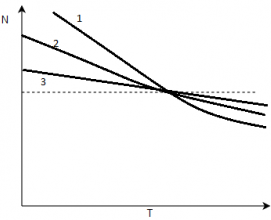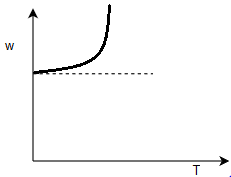Courses

# Test: Operating Characteristics Of DC Motors

## 10 Questions MCQ Test Electrical Machines | Test: Operating Characteristics Of DC Motors

Description
This mock test of Test: Operating Characteristics Of DC Motors for Electrical Engineering (EE) helps you for every Electrical Engineering (EE) entrance exam. This contains 10 Multiple Choice Questions for Electrical Engineering (EE) Test: Operating Characteristics Of DC Motors (mcq) to study with solutions a complete question bank. The solved questions answers in this Test: Operating Characteristics Of DC Motors quiz give you a good mix of easy questions and tough questions. Electrical Engineering (EE) students definitely take this Test: Operating Characteristics Of DC Motors exercise for a better result in the exam. You can find other Test: Operating Characteristics Of DC Motors extra questions, long questions & short questions for Electrical Engineering (EE) on EduRev as well by searching above.
QUESTION: 1

### Mark the correct option which affects the terminal voltage of a dc shunt motor.

Solution:

If the dc motor has compensating winding, flux will remain constant regardless of load.

QUESTION: 2

### For a dc shunt motor of 5 kW, running at 1000 rpm, the induced torque will be

Solution:

Torque = Power/(speed in rev/s)
= 5000/(2*pi*1000/60)
= 47.76 N.

QUESTION: 3

### The flux and the internally generated voltage of a dc machine is a ________ function of its magneto-motive force.

Solution:

The flux and induced emf are non linear function of its mmf.

QUESTION: 4

It is advised not to run dc series motor with no load. Why?

Solution:

If the torque is zero in dc series motor then the speed will be infinite causing the damage of the machine permanently.

QUESTION: 5

Choose the best option which identifies about the below characteristics.​Solution:

Shunt machine has almost constant speed while the series varies as inverse relation with torque.

QUESTION: 6

The speed torque of the differential compound dc motor is shown below. What conclusions can be made?​Solution:

This is basically an unstable scenario which makes the machine unable to be used.

QUESTION: 7

It is impossible to start a differential compounded dc motor.

Solution:

It is so because of the unstable speed and torque characteristics. At starting, armature current and series field current are high. Since the series flux subtracts from shunt flux, series flux actually reverses the magnetic polarity.This motor will typically be still or turn slowly in wrong direction while burning up because of excessive current.

QUESTION: 8

A student is given a differential compound motor and he has been asked to make it start. How will he try?

Solution:

At starting , the flux should be rated so that there is no abnormal situation arise.

QUESTION: 9

For a 100 hp 250 V, compound dc motor with compensating winding has a field current of 5 A to produce a voltage of 250 V at 1200 rpm. What will be the shunt field current of this machine at no load?

Solution:

At no load, armature current is zero, so the internal generated voltage is 250 V. So 5 A will be the shunt field current at no load.

QUESTION: 10

A dc shunt motor is connected to the source through 3-point starter. Suddenly if we starter handle is moved fastly from off to on position, then

Solution:

As the starter is placed very rapidly from off to on the resistance seen by the machine will be very less and it will draw a large starting current.